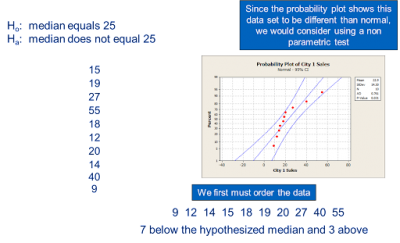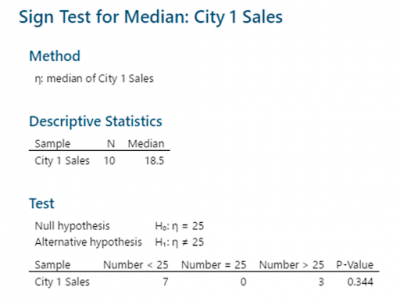If your data is not normally distributed, you may not be able to use parametric hypothesis tests. The use of nonparametric tests like the 1-sample sign test may still allow you to reach conclusions about your data.

## Overview: What is a 1-sample sign test?

Many hypothesis tests strive to draw conclusions about continuous data means. One limitation is the assumption the data comes from a specific population distribution, commonly a normal distribution. For example, if you are interested in determining whether a single sample target mean of 25 minutes comes from a normally distributed population with a mean of 25 minutes, you would use a 1-sample t test.

But, what if your sample data does not conform to the underlying assumption of normality? You might not want to use the parametric 1-sample t test. Instead, you might decide to use a nonparametric test such as a 1-sample sign test. In this case, you would be testing whether the sample median comes from a population with the same median.

As in all hypothesis tests, you start with a null and alternative hypothesis. In the case of the 1-sample sign test, the null hypothesis would be written as: Ho: the median equals some target value. The alternative hypothesis would be written as: Ha: the median does not equal the target value.

While the 1-sample sign test does not have an assumption of a specific probability distribution, there are still some assumptions. The primary one is that the distribution has a single hump and if comparing two or more samples, the shape of the distributions should be approximately the same and the samples are independent.

The output of the test results would provide a p-value which can be used to either reject the null or fail to reject the null.

## An industry example of a 1-sample sign test

The Six Sigma Black Belt wanted to determine whether the median sales of the Miami office was equal to the target of \$25,000. Here is the data and results of her analysis.With a p-value of 0.344, you would not reject the null and therefore conclude the median sales of the sample comes from a population with a median of \$25,000.

### What is the difference between a 1-sample t test and a 1-sample sign test?

The 1-sample t test is a parametric test seeking to conclude something about population means while the 1-sample sign test is a nonparametric test used to conclude something about population medians

### What is the null hypothesis for the 1-sample sign test?

The null hypothesis for a 1-sample sign test is written as: Ho: the median equals xxx (some selected value)

### When should I use a 1-sample sign test?

You will use the 1-sample sign test if you want to know something about population medians or you fail the assumption of normality when attempting to use a parametric hypothesis test.